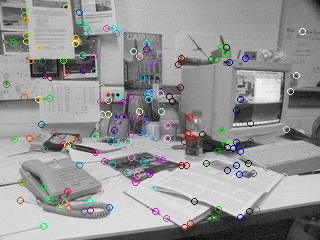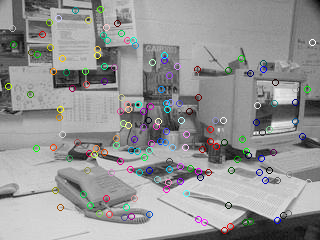## Example of finding the fundamental matrix using RANSAC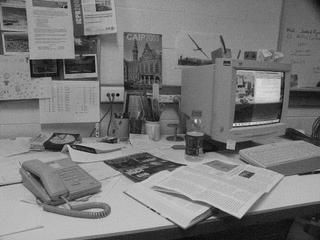im1.jpgim2.jpg
```    % Parameters
dmax = 50;      % Maximum search distance for matching
w = 11;         % Window size for correlation matching

% Find 100 strongest Harris corners in image1 and image2
show(im1,1), hold on, plot(c1,r1,'r+');

show(im2,2), hold on, plot(c2,r2,'r+');
```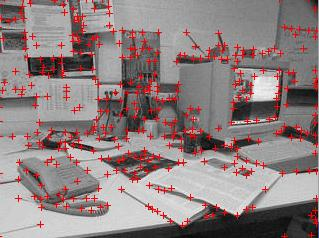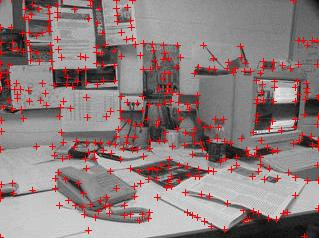```
[m1,m2] = matchbycorrelation(im1, [r1';c1'], im2, [r2';c2'], w, dmax);

% Display putative matches
show(im1,3), set(3,'name','Putative matches'), hold on
for n = 1:length(m1);
line([m1(2,n) m2(2,n)], [m1(1,n) m2(1,n)])
end

``````    % Assemble homogeneous feature coordinates for fitting of the
% fundamental matrix, note that [x,y] corresponds to [col, row]
x1 = [m1(2,:); m1(1,:); ones(1,length(m1))];
x2 = [m2(2,:); m2(1,:); ones(1,length(m1))];

t = .001;  % Distance threshold for deciding outliers
[F, inliers] = ransacfitfundmatrix(x1, x2, t);

% Display both images overlayed with inlying matched feature points
show(double(im1)+double(im2),4), set(4,'name','Inlying matches'), hold on
plot(m1(2,inliers),m1(1,inliers),'r+');
plot(m2(2,inliers),m2(1,inliers),'g+');

for n = inliers
line([m1(2,n) m2(2,n)], [m1(1,n) m2(1,n)],'color',[0 0 1])
end
``````    % Step through each matched pair of points and display the
% corresponding epipolar lines on the two images.

l2 = F*x1;    % Epipolar lines in image2
l1 = F'*x2;   % Epipolar lines in image1

for n = inliers
figure(1), clf, show(im1,1), hold on, plot(x1(1,n),x1(2,n),'r+');
hline(l1(:,n));
figure(2), clf, show(im2,2), hold on, plot(x2(1,n),x2(2,n),'r+');
hline(l2(:,n));
fprintf('hit any key to see next point\r'); pause
end
```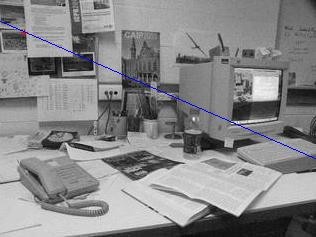Here's another way to visualize the matches suggested by José L. Crespo which works rather nicely. It displays each image with the putative matches marked as circles with different colors. Using the colorcube map produces different colors for nearby points allowing them to be distinguished from each other.

```   col=colormap(colorcube(size(m2,2)));
figure(1), imshow(im1,[]), hold on
scatter(m1(2,:),m1(1,:),[],col);
hold off

figure(2), imshow(im2,[]), hold on
scatter(m2(2,:),m2(1,:),[],col);
hold off
```